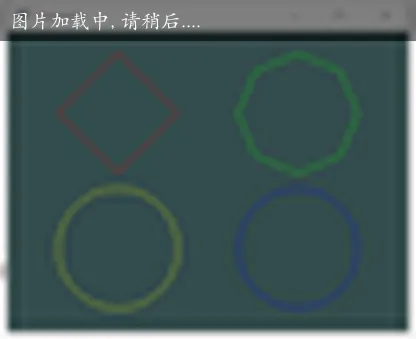## 几何着色器入门

``````layout(points) in;
``````

• points - GL_POINTS (1 vertex)
• lines - GL_LINES, GL_LINE_STRIP, GL_LINE_LIST (2 vertices)
• triangles - GL_TRIANGLES, GL_TRIANGLE_STRIP, GL_TRIANGLE_FAN (3 vertices)

### 输出类型

``````layout(line_strip, max_vertices = 2) out;
``````

• points
• line_strip
• triangle_strip

### 顶点输入

``````in gl_PerVertex
{
vec4 gl_Position;
float gl_PointSize;
float gl_ClipDistance[];
} gl_in[];
``````

### 顶点输出

``````void main() {
gl_Position = gl_in.gl_Position + vec4(-0.1, 0.0, 0.0, 0.0);
EmitVertex();

gl_Position = gl_in.gl_Position + vec4(0.1, 0.0, 0.0, 0.0);
EmitVertex();

EndPrimitive();
}
``````

## 动态生成几何图形

``````void createGeometryShader() {
layout(points) in;
layout(line_strip, max_vertices = 64) out;

in vec3 vColor[]; // Output from vertex shader for each vertex
in float vSides[];

out vec3 fColor; // Output to fragment shader

const float PI = 3.1415926;

void main() {
fColor = vColor; // Point has only one vertex

//gl_Position = gl_in.gl_Position + vec4(-0.1, 0.0, 0.0, 0.0);
//EmitVertex();

//gl_Position = gl_in.gl_Position + vec4(0.1, 0.0, 0.0, 0.0);
//EmitVertex();

for (int i = 0; i <= vSides; i++) {
// Angle between each side in radians
float ang = PI * 2.0 / vSides * i;

// Offset from center of point (0.3 to accomodate for aspect ratio)
vec4 offset = vec4(cos(ang) * 0.3, -sin(ang) * 0.4, 0.0, 0.0);
gl_Position = gl_in.gl_Position + offset;

EmitVertex();
}

EndPrimitive();
}
);